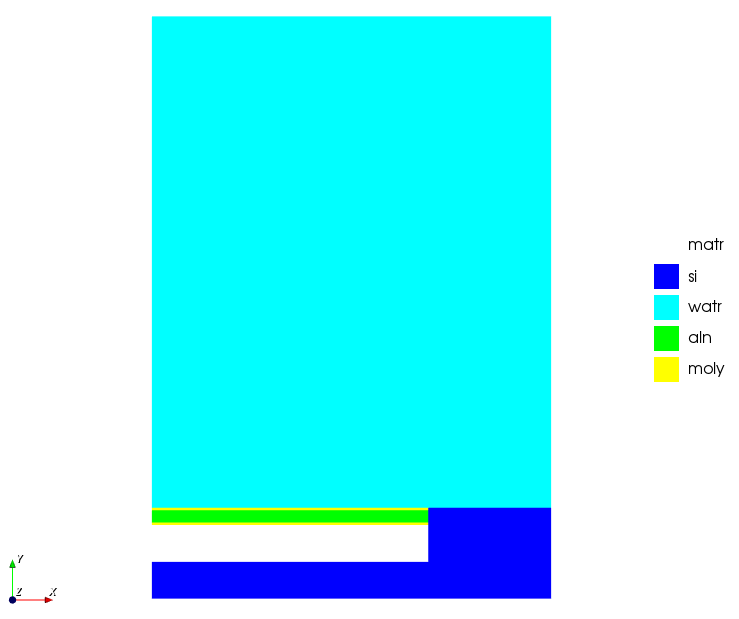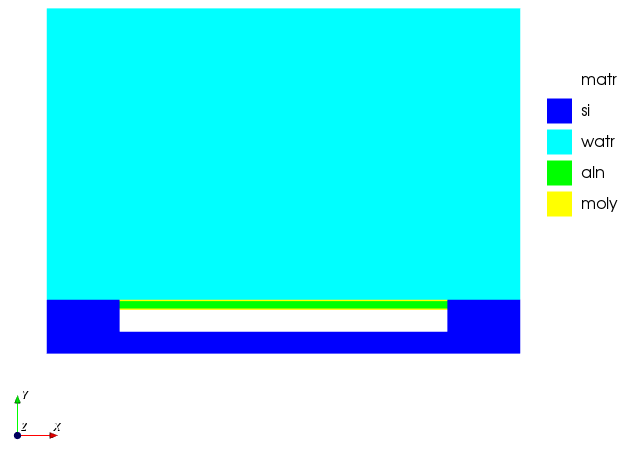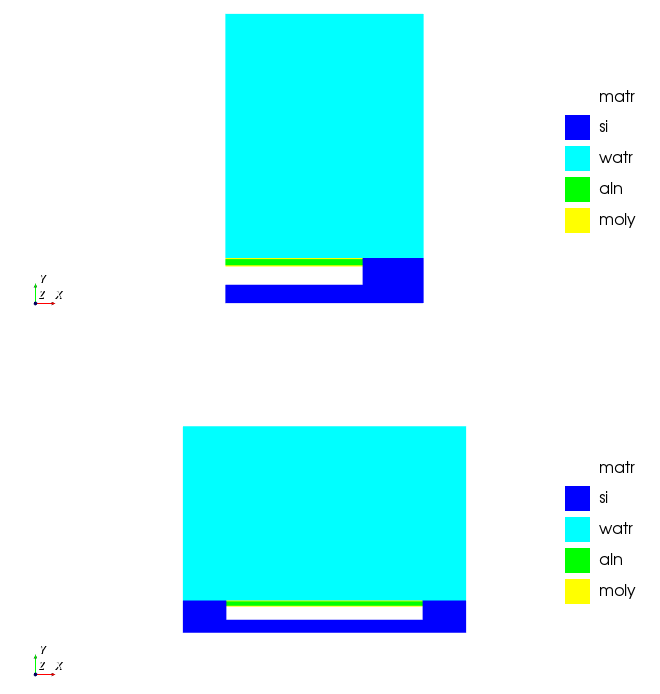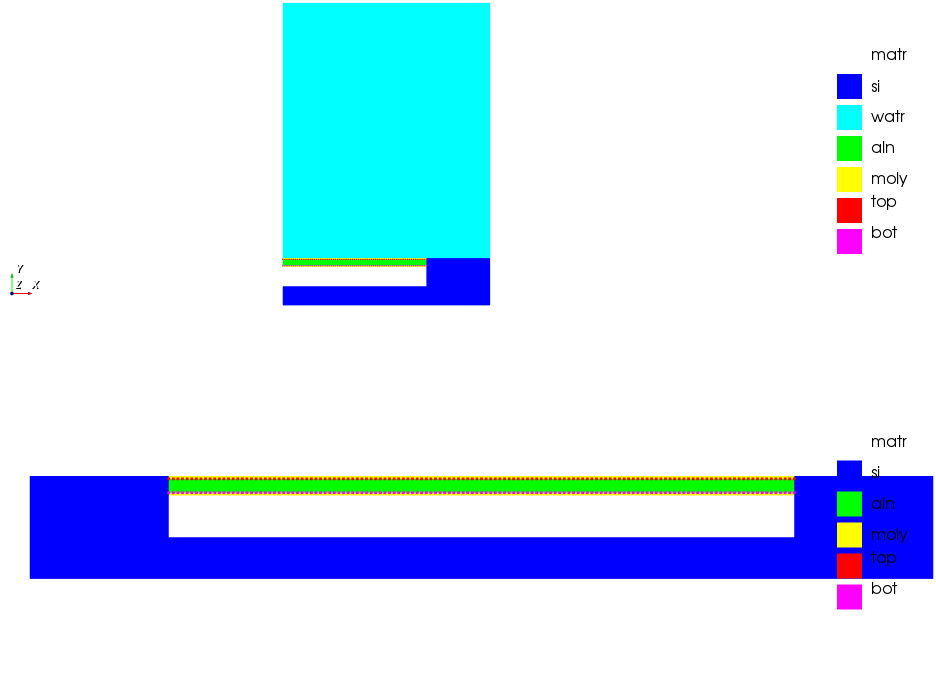# GRPH command (plotting materials)

The GRPH command provides an interactive graphics display of computational model and results of analysis. Graphical information allows you to confirm that it has been built as intended and the identification of any problems with the input file. By displaying any fields of interest (these need to be requested) progress can be viewed as the model is running, if everything is set up and used correctly. These capabilities are accessible through the GRPH PLOT options.

OnScale Lab also has the option for interactive run time graphics that allows users to rotate their model in full 3D, which is very useful in the model building/pre-processing stage.

The following are examples using the PMUT 3D example:

• The elements assigned material properties can be displayed using the following code snippet:
```grph
plot matr     end term```• If the model is symmetrical along any of the x, y or z axis it is recommended to only model half of the geometry and apply symmetry later. This is what will be done to the example above.
`grph     mirr x on      plot matr     endterm`• It is possible to view both of these material plots at the same time using the sub command 'nvew'
`grph      nvew 2 1     plot matr     mirr x on      plot matr     endterm`• Another common use of the GRPH command when applying electrodes to any model is to view that they have been placed in the correct locations this can be done using 'plot piez'. This would be done after defining the electrodes and would follow the PIEZ command. This example will also sow how to plot the device without the watr utilising the keypoint
`grph      nvew 2 1     plot matr piez              /* '&' plots electrode and material plot on the same plot     mirr x on      plot matr piez j \$j1 \$j6    /* This plots everything from the 1st keypoint to the 6th in the y (j) direction      endterm`This is just a simple introduction into some of the more basic and common elements of plotting done on OnScale. The GRPH command has an extensive list of subcommands that can be found in the dedicated help found in the help tab of the application ribbon.